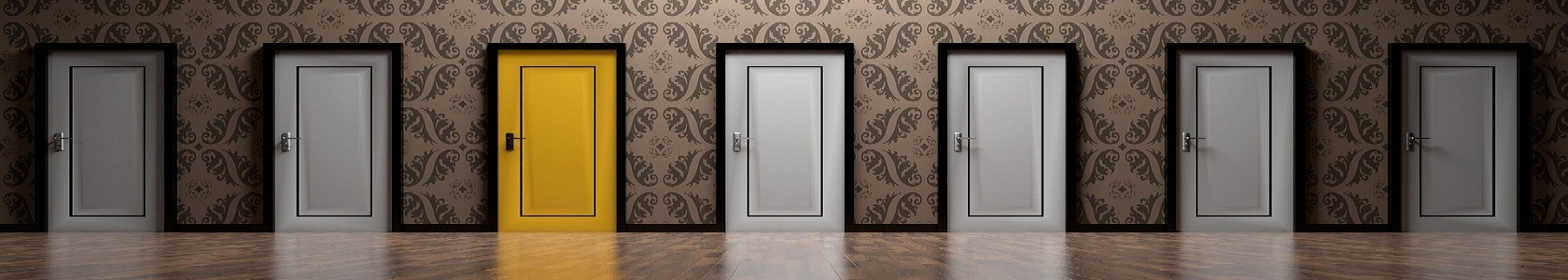# Nursing quiz

##### Nursing Research Quiz - 61 2 3 4 5 6 7 8 9 10 11 12 13 14 15 16 17 18 19 20 21 22 23 24 25 26 27 28 29 30

#### Nursing Research Quiz - 6

##### epidemiology

1. All of the following diagrams can be draw from quantitative data, except:

A. Frequency curve

B. Scatter diagram

B. Flow diagram

D. Histogram

2. In a community correlation between infant mortality and socioeconomic status is:

A. r = +1 (Strong positive correlation)

B. r = -1 (strong negative correlation)

C. r = -0.8 (moderate negative correlation)

D. r = +0.22 (strong positive correlation)

3. Best graph for demonstration of relationship between ages and weight is:

A. Bar Diagram

B. Histogram

C. Scatter

D. Pie diagram

4. Age and sex representation is best represented by:

A. Histogram

B. Pie chart

C. Bar diagram

D. Sketch of photos

5. Quantitative data can be best represented by:

A. Pie chart

B. Pictogram

C. Histogram

D. Bar diagram

6. Percentage of data can be shown in:

A. Graph presentation

B. Pie chart

C. Bar diagram

D. Histogram

7. The average berth weights in a hospital are to be demonstrated by statistical representation. This is best done by:

A. Frequency curve

B. Pictogram

C. Histogram

D. Bar diagram

8. In a random sample taken for a population, the median is higher than:

A. 25

B. 50

C. 67

D. 100

9. For determination of which statistical parameter requires quantities to be arranged in an ascending or descending order?

A. Mean

B. Median

C. Mode

D. SD

10. In a population of 100 females in the age group of 15-45, the mean systolic BP was found to be 120. In a normal curve distribution, the number of people who would have an average BP above 120 will be:

A. 25

B. 50

C. 75

D. 95

11. The two important values necessary for describing the frequency distribution of a series of observation are:

A. Mean and standard deviation

B. Mean and range

C. Median and range

D. Median and standard deviation

12. Arrangement of values in a serial order is to determine:

A. Mean

B. Mode

C. Median

D. Range

13. A scatter diagram shows:

A. Trends of events with the passage of time

B. Relationship between two variables

C. Proportion of an event

D. Positive relationship between two variables

14. In 11 babies born in a hospital is 5 above 2.5 kg and 5 below 2.5 kg. The value 2.5 is:

A. Median average

B. Mode average

C. Geometrical mean

D. Arithmetic average

15. Relationship between two variables can be presented by:

A. Pie diagram

B. Scatter diagram

C. Bar diagram

D. Histogram